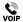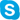# ReVerie guide to calculating a force from a coefficient. Scaling speeds.

## Plus conversion from force in Newton’s to Kilograms.

ReVerie guide to calculating a force from a coefficient. Scaling speeds.
Plus conversion from force in Newton’s to Kilograms.
Lift figures should be expressed as a negative if providing downforce. The same calculation is used for both lift and drag calculations.

## To calculate from the coefficient:

Force (N) = Half x Air Density x Frontal Area (M2) x Coefficient x Velocity Squared (MPS2)
Force (N) = ½ x 1.225 x Frontal Area x Coefficient x Velocity x Velocity

We will use the coefficient of lift from one of the ReVerie modified Noble M400 tests -0.554, 44.704 meters per second as the velocity which is 100 miles per hour plus a frontal area of 1.87M^2.

Force (N) = ½ x 1.225 x 1.87 x -0.554 X 44.7042
Force (N) = -1329.12

Convert the force (N) into kilograms: Kilograms = Force (N) ÷ 9.81
Kilograms = -1329.12 ÷ 9.81
Kilograms = -135.486

For example:
The ReVerie modified Noble M400 has -1329.12 Newtons of lift (downforce) which is 135.486 kilograms of downforce at 100MPH. The lift and drag coefficients are speed independent. You can obtain the figures in Newtons (N) for any velocity by using the conversion below.

To convert miles per hour MPH into meters per second MPS:
1 MPS = 2.23693629 Miles per Hour
MPS = 100 ÷ 2.23693629
MPS = 44.704
100 miles per hour is equal to 44.704 meters per second.

New Force (N) = Original Force (N) x (New Speed2 (MPH) ÷ Data Speed2 (MPH))
New Force = 1329.12 x ((150 x 150) ÷ (100 x 100))
New Force = 1329.12 x (22500 ÷ 10000)
New Force = 1329.12 x 2.25
New Force = 2990.52
Above we have used the ReVerie modified Noble M400 downforce at 100 MPH and scaled it to 150 MPH.
This would also work for scaling forces converted into kilograms.

To convert drag into BHP absorbed (Drag = 100N in this Equation):
BHPAbs = (2 x Drag (N) x Speed MPS) ÷ 1500
BHPAbs = (2 x 100 x 44.704) ÷ 1500
BHPAbs = 8940.8 ÷ 1500
BHPAbs = 5.96

If  you have a figure for the drag coefficient of a car then you can calculate the BHP absorbed at any speed, e.g Cd = 0.4, frontal area = 2m^2, speed = 100mph (44.7m/s) then:

BHPabs = (Cd x area m2 x speed^3 m/s) ÷ 1225
= (0.4 x 2 x 44.7^3) ÷ 1225 [NB 44.7^3 means 44.7 x 44.7 x 44.7]
= 58.3 BHP

NB - to calculate the power absorbed by a wing you need to know its drag coefficient and its PLAN area, which is the reference area used with wings. Or more simply, use drag force data and the calculation above to convert that to BHP absorbed.+44(0)1206 86 81 44
+44 (0)1206 86 66 63+44(0)2081 235997 / reverie_composites